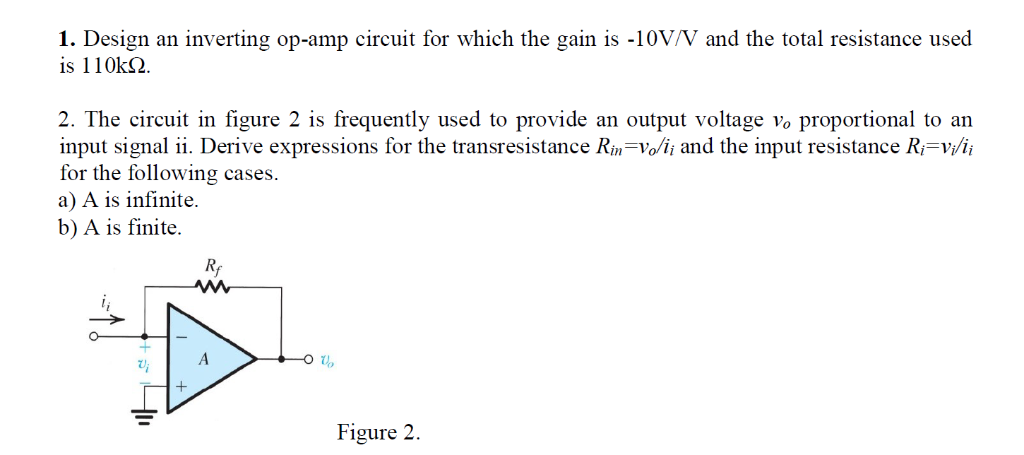# Solved: 1. Design Is 110k2 An Inverting Op-amp Circuit For Which The Gain Is -10V/V And The Total Resistance Used Provide Proportional To An 2. The Circuit In Figure 2 Is Frequently Used To Input Sign

By |1. Design is 110k2 an inverting op-amp circuit for which the gain is -10V/V and the total resistance used provide proportional to an 2. The circuit in figure 2 is frequently used to input signal ii. Derive expressions for the transresistance Rin=Vo/ii and the input resistance R=vi/i for the following cases a) A is infinite b) A is finite an output voltage vo R A Figure 2